# What is an Electric Field?An electric field is a field or space around an electrically charged object where any other electrically charged object will experience a force.

An electric field is measured by a term known as electric field intensity. If we place a positive unit charge near a positively charged object, the positive unit charge will experience a repulsive force. Due to this force, the positive unit charge will move away from the said charged object. The imaginary line through which the unit positive charge moves, is known as line of force.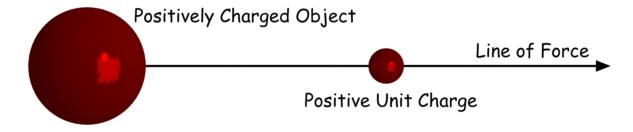Similarly, if we place a positive unit in the field of a negatively charged object, the unit positive charge will experience an attractive force. Due to this force, the unit positive charge will come closer to the said negatively charged object. In that case, line through which the positive unit charge moves, is called line of force.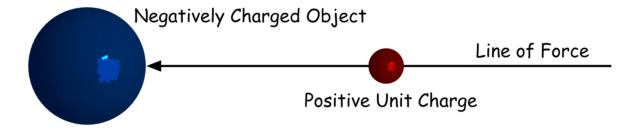We can place a unit positive anywhere surround the positively charged object and each position where we place it, the unit positive charge follows a separate line force to move. Hence, we can say, the lines of force get radiated or come out from this charged object.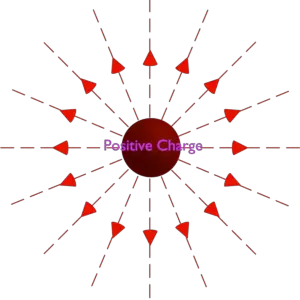But for a negatively charged object, these lines of force come into this negatively charged object.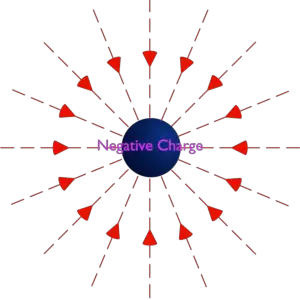## What is the value of electric field?

Electric field at a point in the space is measured as the force acting on the unit positive charge at that point. When a charged object enters the electric field of another charged object, it experiences a force as per Coulomb’s law.

Let us take a charged object, of charge Q1 Coulomb. Let us place one unit positive charge at r meter away from the center of Q1.So, as per Coulomb’s law, the force experienced by the unit positive charge is,Here, we have considered that the medium is air or vacuum in which we placed both charge Q1 and unit positive charge. The force experienced by the unit positive charge is the measurement of the electric field of Q1 at the point where we have placed the unit positive charge. This force vector is denoted by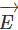. The termis known as electric field intensity or electric field strength.
Now, we place an object of charge Q2 coulombs at same point where, unit positive charge was placed.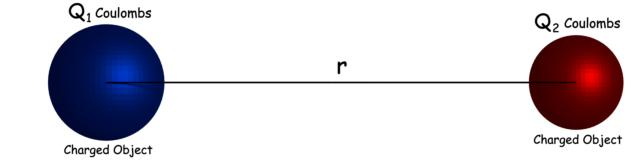Two positively charged objects repel each other, two negatively charged objects repel each other and two oppositely charged objects attract each other with force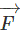.

This attraction or repulsion forcecan be written as.So, the vector of electric field, determines how strongly an electric charge is repulsed or attracted by the charge which has created the electric field.

## What is the direction of electric field?

When we place the imaginary unit positive charge in an electric field, the unit positive charge starts moving due to electrostatic force of the field. The unit charge either comes closer or goes far, depending upon the nature of the charge, by which the field is created. The direction of the movement of the unit positive charge in the field is considered as the direction of the electric field. So, an electric field is a vector quantity.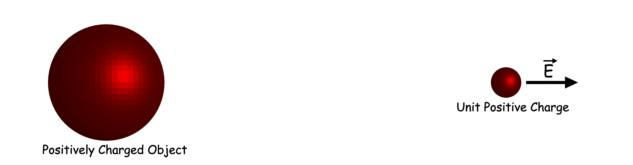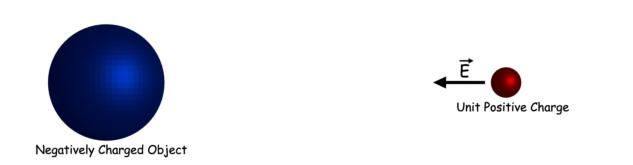## Static Electric Field

We know there are two types of charge present in nature (i) positive and (ii) negative charge. In positive charge, there is mainly deficiency of electrons and in a negative charge, there are excess of electrons. Now, we can simply understand the concepts of charge from a very basic example. Take a dry comb, comb your hair (which should be dry) two to three times, now take that comb near tiny pieces of paper, you will see that the paper pieces are getting attracted to the comb. This is the very basic example of electric charge and static electric field. Due to friction, there is a movement of electrons between comb and hair, so one of them gets positively charged and another one gets negatively charged and as the paper is neutral (i.e. not charged) they get attracted to the comb.

So, we can see that there is an attraction force works between a charged particle and neutral particle, it has been seen further that there is repulsion between two same charged particles and attraction between two oppositely charged particles. This happens due to the field created by a particle. This can be understood if we imagine a glowing bulb, the bulb can be taken as the charge and the visible light can be compared to static electric field, the characteristic of the field is similar to the light in the sense that the intensity of the field is greater near the source and it fades as we move further from the source. Now from another point of view, we can say that static electric field is nothing but an intense space, in terms of power where work is done or needed to be done upon in presence of an electrically charged particle depending on the nature of the charged particle.

Want To Learn Faster? 🎓
Get electrical articles delivered to your inbox every week.
No credit card required—it’s 100% free.